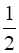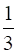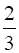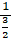### Mark the correct answer in the following questions. If the refractive index of glass with respect to air is 3/2, what is the refractive index of air with respect to glass?

Question 6.

Mark the correct answer in the following questions.

If the refractive index of glass with respect to air is 3/2, what is the refractive index of air with respect to glass?
A.B. 3

C.D.Answer:

Since refractive index of medium 2 with respect to medium 1 = 1/refractive index of medium 1 with respect to refractive index of medium 2. So if refractive index of glass with respect to air is 3/2 so refractive index of air with respect to glass ==